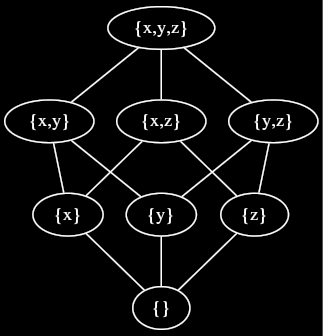# Poset: Exercises

Try these exercises to test your poset knowledge.

Imagine a company with five distinct roles: CEO, marketing manager, marketer, IT manager, and IT worker. At this company, if the CEO gives an order, everyone else must follow it. If an order comes from an IT manager, only IT workers are obligated to follow it. Similarly, if an order comes from a marketing manager, only marketers are obligated to follow it. Nobody is obligated to follow orders from marketers or IT workers.

Do the workers at this company form a poset under the “obligated to follow orders from” relation?

While not technically a poset due to its lack of reflexivity, it is pretty close. It is actually a strict ordering, whose underlying partial order could be obtained by making the reasonable assumption that each worker will follow her own orders.

# Bag Inclusion

We can define a notion of power sets for bags as follows. Let $$X$$ be a set, then we use $$\mathcal{M}(X)$$ to denote the set of all bags containing elements of $$X$$. Let $$A \in \mathcal{M}(X)$$. The multiplicity function of $$A$$, $$1_A : X \rightarrow \mathbb N$$ maps each element of $$X$$ to the number of times that element occurs in $$A$$. We can use multiplicity functions to define a inclusion relation $$\subseteq$$ for bags. For $$A, B \in \mathcal M(X)$$, we write $$A \subseteq B$$ whenever for all $$x \in X$$, $$1_A(x) \leq 1_B(x)$$.

Does $$\mathcal{M}(X)$$ form a poset under the bag inclusion relation $$\subseteq$$? If so, prove it. Otherwise, show that it does not satisfy one of the three poset properties.

# Duality

Give the dual of the following proposition.

For all posets $$P$$ and all $$p, q \in P$$, $$q \prec p$$ implies that $$\{ r \in P~|~r \leq p \}$$ is a superset of $$\{ r \in P~|~r \leq q\}$$.

For all posets $$P$$ and all $$p, q \in P$$, $$q \succ p$$ implies that $$\{ r \in P~|~r \geq p \}$$ is a superset of $$\{ r \in P~|~r \geq q\}$$ (where $$q \succ p$$ means $$p \prec q$$).

# Hasse diagrams

Let $$X = \{ x, y, z \}$$. Draw a Hasse diagram for the poset $$\langle \mathcal P(X), \subseteq \rangle$$ of the power set of $$X$$ ordered by inclusion.<div><div>

comment: dot source:

digraph G { node = 0.1, height = 0.1 edge = “none” e = “{}” x = “{x}” y = “{y}” z = “{z}” xy = “{x,y}” xz = “{x,z}” yz = “{y,z}” xyz = “{x,y,z}”

rankdir = BT; e → x e → y e → z x → xy x → xz y → xy y → yz z → xz z → yz xy → xyz xz → xyz yz → xyz } <div>%%

%%

# Hasse diagrams (encore)

Is it possible to draw a Hasse diagram for any poset?

Note that our description of Hasse diagrams made use of the covers relation $$\prec$$. The covers relation, however, is not adequate to describe the structure of many posets. Consider the poset $$\langle \mathbb R, \leq \rangle$$ of the real numbers ordered by the standard comparison $$\leq$$. We have $$0 < 1$$, but how would we convey that with a Hasse diagram? The problem is that $$0$$ has no covers, even though it is not a maximal element in $$\mathbb R$$. In fact, for any $$x \in \mathbb R$$ such that $$x > 0$$, we can find a $$y \in \mathbb R$$ such that $$0 < y < x$$. This “infinite density” of $$\mathbb R$$ makes it impossible to depict using a Hasse diagram.

Parents:

• Does the first question seem a bit much of a ‘gotcha’? I was slightly annoyed I got it wrong despite being quite capable of working with posets. XD

I suppose the way it is asked is completely valid, and will drive home the fact that relations have to be reflexive and illustrates very well what would be necessary to get a valid order.

What are other people’s thoughts?

(Maybe it will be better once it isn’t the only question, nor the first?).

• I proposed an edit to fix these 2 issues (in the 1st example):

1) The answer claims there is no anti-symmetry, which is mistaken. In particular, if something breaks anti-symmetry, it cannot be a valid strict ordering (which is another claim from the answer).

2) This is less serious, but saying “everyone must follow orders that come from the CEO” in natural language is unclear on whether the CEO follows orders from himself.

• Thanks Chris. Edit accepted.

• Not clear what this means?